Hello folks, it’s been a while! First, there was Wappu (see here for some info) – kind of a big deal for tech students and alumni alike – after which I took a week-long holiday in my home region. Anyway, I’m safely and happily back now, working on model order reduction for electrical machines. In other words, I’m trying to develop a computationally faster alternative to time-stepping finite element analysis (FEM).

As you may know, the (most common) idea of FEM is the express the magnetic flux density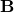with the so-called vector potential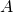through the relationship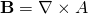,

and then solveat a number of coordinates called nodes. This, in the simplest case, yields a matrix equation of type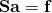,

where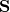is the so-called stiffness matrix and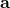is an unknown vector containing the nodal potentials. Finally,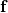is a load or source vector, usually containing either currents or voltages depending on the problem formulation.

Since I’m interested in an actually rotating machine, everything will be time-dependent. In other words, we have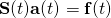The source vector is not problematic, since we know what it looks like by definition (it’s the source, i.e. something we purposefully excite the system with). Moreover, the stiffness matrix can split into it time-dependent and independent components, i.e.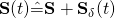,

but that’s not a problem either.

No, it’s the time-dependent vector potential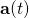that’s causing problems. Even in this simple case, we would have to solve the system at many separate instantsto approximate howbehaves in time.

Moreover, we often have conducting components somewhere in the problem domain. These conductors can then develop eddy currents. These currents, although occasionally cool, have the nasty characteristic that they are dependent on the derivative of. Thus, we have to solve a time-dependent differential equation with a suitable time-stepping method.

Even if we didn’t have eddy currents, we are often supplying the machine with a voltage rather than a current source. In this case we will have to couple the system with some circuit equations. And these are again dependent on some time-derivatives, which again requires the use of time-stepping.

The point being, we need to solve a somewhat similar-looking problem again and again. Indeed, something like 400 time-steps per one rotor rotation seems to be the de facto minimum. Morever, several revolutions are typically required to reach steady-state. That alone leaves us with several thousand time-steps, easily.

Now, bear in mind that we have a large number of unknowns inat each step. Something in the order of thousands or tens of thousands is very common (I have gone up to 1.2 million once or twice). Solving a problem of this size thousands of times is not very fast.

Not fast at all.

And this is precisely the problem I’m trying to tackle. The exact how will have to wait for a while, to avoid this post blowing up into unbearable lengths.

For now, enjoy the small teaser below.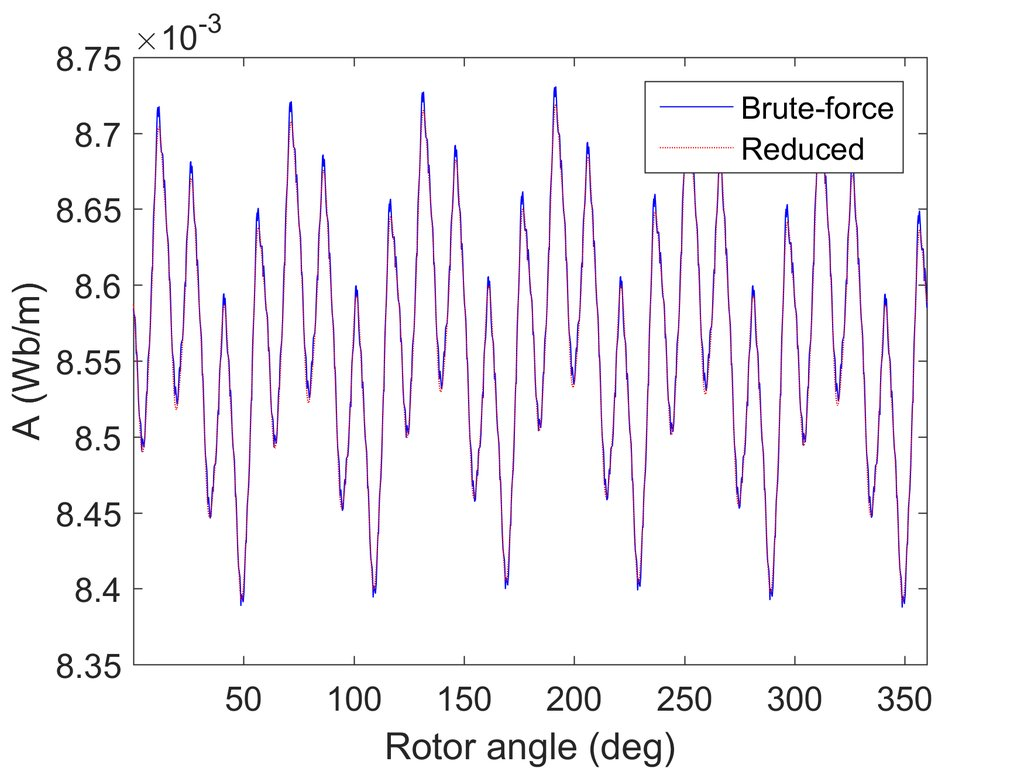Simple time-stepping compared to a reduced-order model.

What you see is the value of the vector potentialat one node on the rotor surface, as the rotor is rotated one full turn. The blue curve has been obtained by computing the full solutionat 1000 different rotor angles. Since there were approximately 1500 nodal potentials, that corresponds to 1.5 million data points.

By contrast, the red curve displays the results from a reduced-order model. This time however, only 22 500 data points were computed, less than 1/60 of the brute-force solution.

Still, the results very accurate, almost impossible to tell apart with naked eye.

Now that’s cool, ain’t it?

But how?

Need help with electric motor design or design software? Let's get in touch - satisfaction guaranteed!Making time-stepping FEM faster